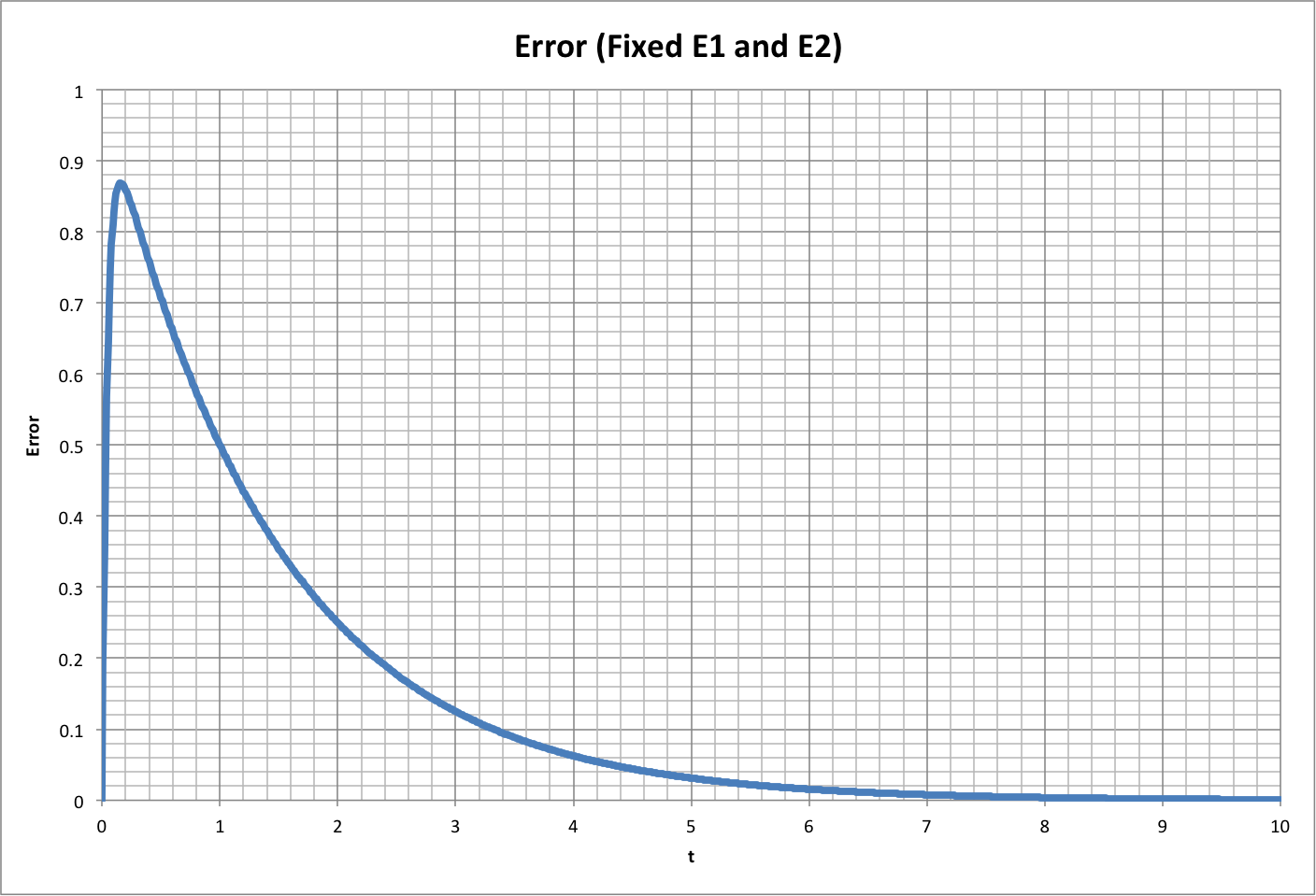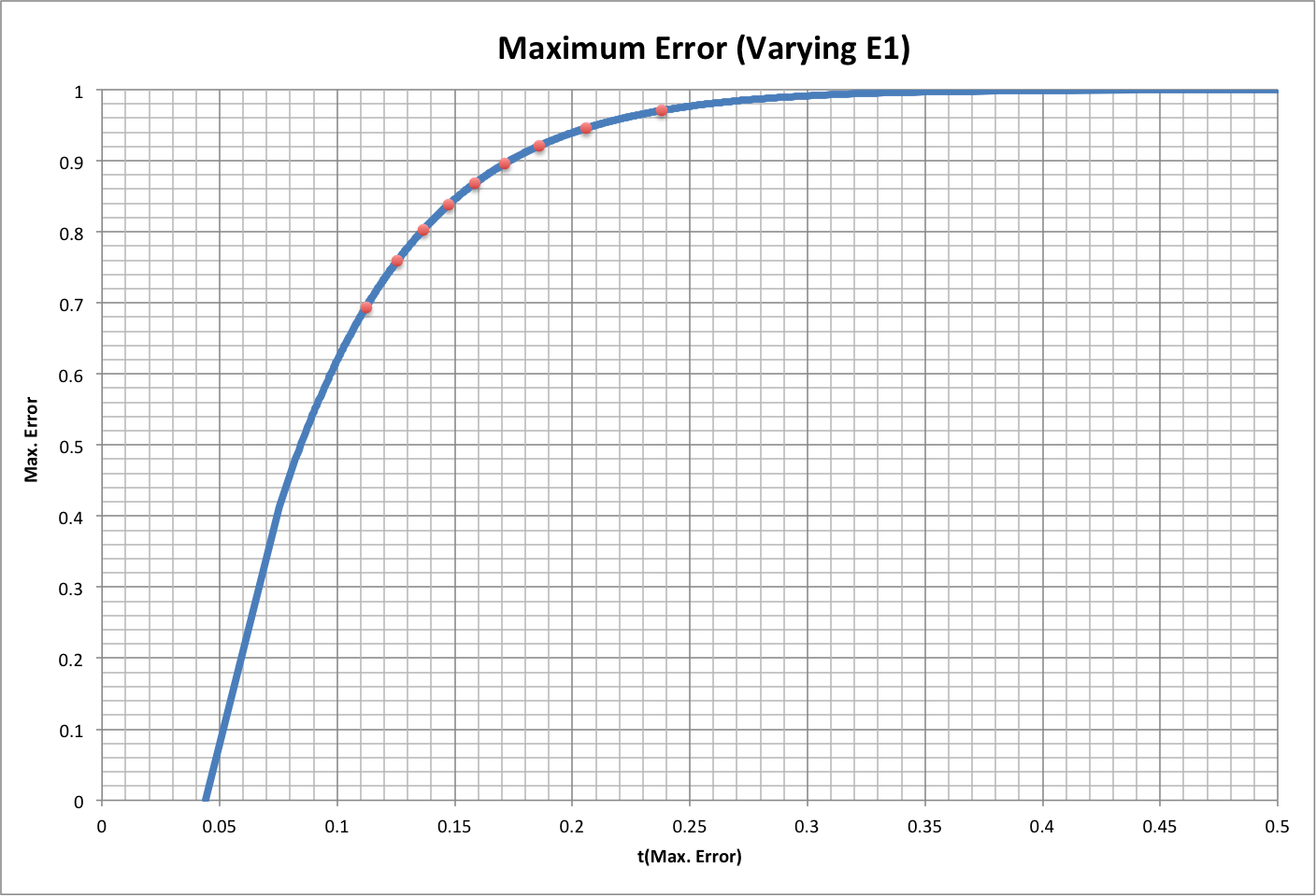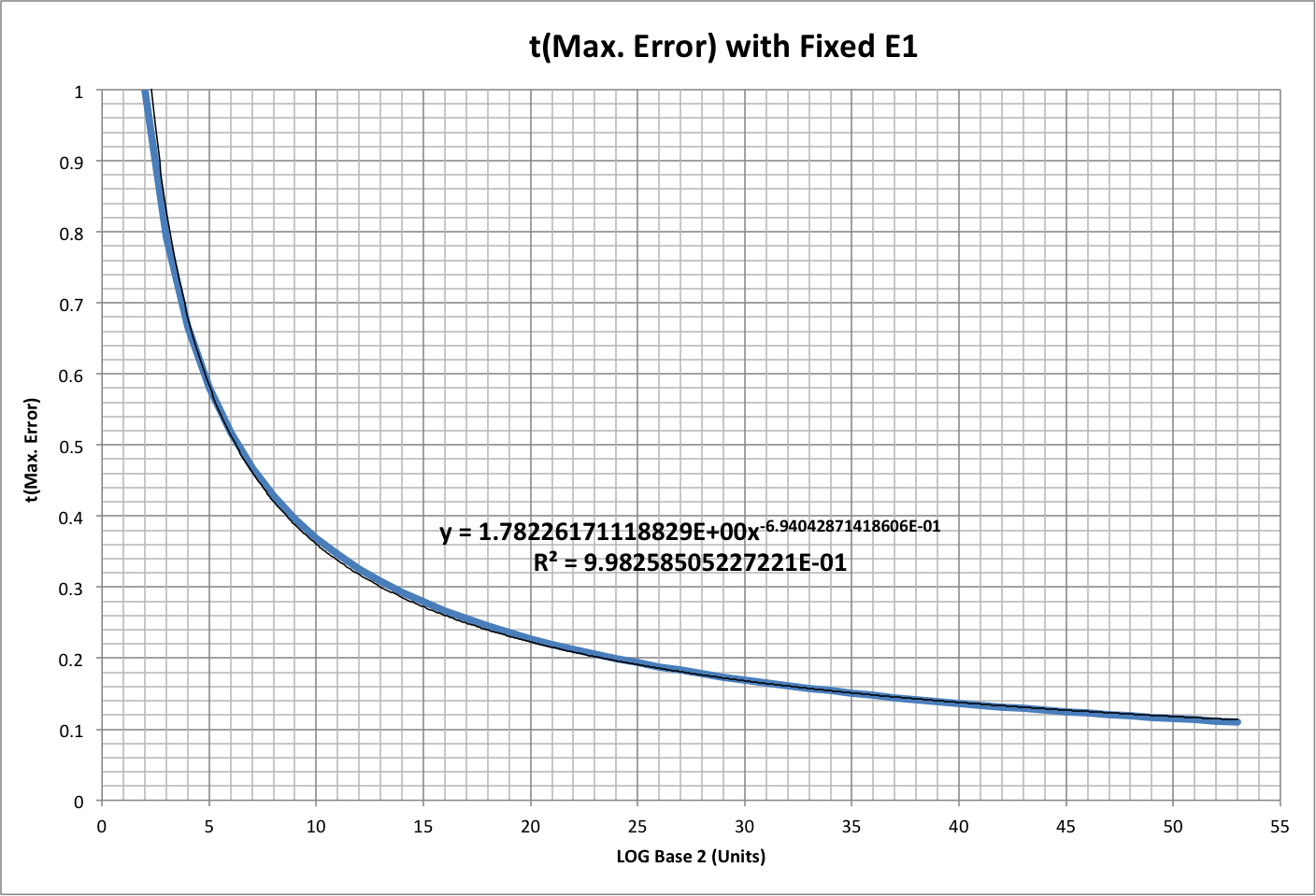# Maximum Error

See Normal Completion for background.

For a given task, the difference between the quality Q1 achieved over time with efficiency E1 and the quality Q2 achieved over time with expected efficiency E2 is the "error":

Error = Q2 - Q1 = (1 - E1)t - (1 - E2)t

Here, t is in units of best-case time to perform the task. Note that this is the negative of the traditional definition of error, Q1 - Q2, so that the value is positive.

The maximum measurable quality Qmax is defined by the resolution, or Units composing the task:

Qmax = 1 - 1 / Units

Setting E2 = Qmax ensures that Qmax will be achieved at t = 1 with the expected efficiency.

The following graph shows how Error varies over time for an average person (E1 = 0.5) and Units equal to the world's population in 2013 (P2013  = 7.1 E+09):This pattern is common to all values of E1 and E2, with an early peak, an error equal to E2 - E1 at t = 1, and a decay towards zero.

The maximum error occurs at the following time, with the value as calculated by Q2 - Q1 above, and where LN is the natural logarithm:

tMax. Error = LN[LN(1 - E2)/LN(1 - E1)] / LN[(1 - E1) / (1 - E2)]

This time and the maximum error are graphed below for different values of E1 and E2 is the same as above (1 - 1 / P2013). Note that the red dots indicate specific values of E1 (from right to left: 0.1, 0.2, ... 0.9).The following graph shows what happens to the peak when we just vary E2. In this case, E1 = 0.5, and Units are doubled 52 times (starting at 4, from right to left, with each doubling indicated by a red dot). A curve fit is calculated for reference. Note that if E1 was less than 0.5, the peak time could exceed 1.The following graph breaks this down as functions of Units (expressed as the base-2 logarithm). Again, E1 = 0.5, and E2 = 1 - 1 / Units. The top graph shows the peak time (along with a curve fit), and the roll-over graph shows maximum error.See the blog post "Complexity and Error" for a discussion of this topic.

© Copyright 2015 Bradley Jarvis. All rights reserved.